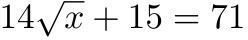# Teaching Math: Fractions and Order of Operations

## Don’t Forget the FractionsIf you are teaching the order of operations to your students, you should also teach them how to do fractions and order of operations. This can be a little challenging because many students have a hard time with fractions. Before you teach this lesson, review adding, subtracting, multiplying, and dividing fractions. Here is a simple lesson plan and free worksheets of order of operations that you can do with your students.

Materials

• paper
• pencil

Introduction

1. Ask students to list the steps to brushing their teeth or sending an email.
2. Write the steps on the board.
3. Ask the students if they could do those things by doing the steps in a different order. Since they will most likely say no, tell them that the same thing needs to happen with math and order of operations.
4. There are a series of steps you must take in order to come up with an answer. Next, review some operations of fractions problems with the students. Here are a few problems you can do:

½ + ¾

5/6 – 1/3

½ * ½

1/8 ÷ 1/3

Allow students a few minutes to work out the problems. This should take no longer than 5 minutes. Let students put the problems on the board when finished. Review the correct way to solve these problems.

Method

1. Write the order of operations on the board – Grouping Symbols, Exponents, Multiplication, Division, Addition, and Subtraction,
2. Tell students to copy it down. Be certain that understand that grouping symbols include (parentheses), {braces}, and [brackets.] Make sure students know all three of the grouping symbols. Also, make sure you tell the students that you do multiplication and division from left to right, as well as addition and subtraction.
3. Keep the problems easy at first and then increase the difficulty of the problems.
4. Do as many problems that you feel are needed. Here are a few problems (increasing by difficulty):

½ + 1/3 * ¾

2/3 + (1/2 * ½) – ¼

{[3/4 – ½] + 5/6}

7/8 ÷1/2 + 2/3 – ¼

½ + ½ * 5/6 – 1/9

After a few problems have been completed, assign students to complete some problems on their own. Give them no more than 10. Write them on the board. Give them at least 20 minutes to complete the problems. Walk around the room to check on students’ progress. When all the students are finished have volunteers write the answers on the board. Tell students to correct their work as you review the problems.

Closure

To finish the lesson, review the order of operations one more time. As homework, give them a worksheet with no more than 15 problems on it to complete, as these take a long time to complete. When the class is just about over (about 5 minutes left), erase the board and tell the students to put everything away except a pencil. Give each student a small slip of paper. Write the following problem on the board and have the students complete it and hand it to you before they leave: [5/6 * 1/2 + 1/3] * ½ + 1/4. By having them complete this problem before they leave you can see if they understood the lesson and can reteach it the next day if necessary.

## Image Credit

Wikimedia Commons – Fractions and Order of Operations – Equation

## This post is part of the series: Making Math Fun

Learn ways to make math an exciting adventure for your students and get the engaged.